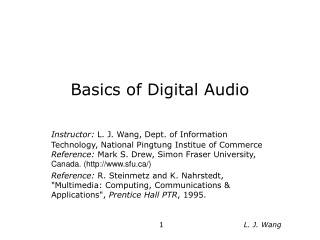DownloadDownload PresentationBasics of Digital Audio

# Basics of Digital Audio

Télécharger la présentation## Basics of Digital Audio

- - - - - - - - - - - - - - - - - - - - - - - - - - - E N D - - - - - - - - - - - - - - - - - - - - - - - - - - -
##### Presentation Transcript

1. Basics of Digital Audio Instructor:L. J. Wang, Dept. of Information Technology, National Pingtung Institue of CommerceReference: Mark S. Drew, Simon Fraser University, Canada. (http://www.sfu.ca/) Reference: R. Steinmetz and K. Nahrstedt, "Multimedia: Computing, Communications & Applications", Prentice Hall PTR, 1995. L. J. Wang

2. Facts about Sound • Sound is a continuous wave that travels through the air. • The wave is made up of pressure differences. • Sound is detected by measuring the pressure level at a location. • Sound waves have normal wave properties (reflection, refraction, diffraction, etc.). L. J. Wang

3. Digitization • Microphones, video cameras produce analog signals (continuous-valued voltages). • To get audio or video into a computer, we must digitize it (convert it into a stream of numbers). • We have to understand discrete sampling (both time and voltage). L. J. Wang

4. Digitization in General (II) • Sampling : • Divide the horizontal axis (the time dimension) into discrete pieces. • Uniform sampling is ubiquitous. • Quantization : • Divide the vertical axis (signal strength) into pieces. • A linear or non-linear function is applied. • 8 bit quantization divides the vertical axis into 256 levels. • 16 bit gives you 65536 levels. L. J. Wang

5. Digitizing Audio • Questions for producing digital audio (Analog-to-Digital Conversion): • How often do you need to sample the signal? • How good is the signal? • How is audio data formatted? L. J. Wang

6. Nyquist Theorem • Nyquist rate -- For good digitization, the sampling rate should be at least twice of the maximum frequency. • Suppose we are sampling a sine wave. How often do we need to sample it to figure out its frequency? L. J. Wang

7. Nyquist Theorem (II) • If we sample at 1 time per cycle, we can think it's a constant. L. J. Wang

8. Nyquist Theorem (III) • If we sample at 1.5 times per cycle, we can think it's a lower frequency sine wave --> Alias. L. J. Wang

9. Signal to Noise Ratio (SNR) • In any analog system, some of the voltage is what you want to measure (signal), and some of it is random fluctuations (noise). • Ratio of the power of the two is called the signal to noise ratio (SNR). SNR is a measure of the quality of the signal. • SNR is usually measured in decibels (dB). L. J. Wang

10. Signal to Quantization Noise Ratio (SQNR) • The precision of the digital audio sample is determined by the number of bits per sample, typically 8 or 16 bits. • The quality of the quantization can be measured by the Signal to Quantization Noise Ratio (SQNR). • The quantization error (or quantization noise) is the difference between the actual value of the analog signal at the sampling time and the nearest quantization interval value. • The largest (worst) quantization error is half of the interval. L. J. Wang

11. Signal to Quantization Noise Ratio (SQNR) (II) • Given N to be the number of bits per sample, the range of the digital signal is - 2 exp (N-1) to 2 exp (N-1). • In other words, each bit adds about 6 dB of resolution, so 16 bits enable a maximum SQNR = 96 dB. L. J. Wang

12. Linear and Non-linear Quantization • Samples are typically stored as raw numbers (linear format ), or as logarithms (u-law (or A-law in Europe)). • Logarithmic quantization approximates perceptual non-uniformity. L. J. Wang

13. Typical Audio Formats • Popular audio file formats include .au (Unix workstations), .aiff (MAC, SGI), .wav (PC, DEC workstations) • A simple and widely used audio compression method is Adaptive Delta Pulse Code Modulation (ADPCM). Based on past samples, it predicts the next sample and encodes the difference between the actual value and the predicted value. L. J. Wang

14. Audio Quality vs. Data Rate • Telephone uses u-law encoding, others use linear. So the dynamic range of digital telephone signals is effectively 13 bits rather than 8 bits. • CD quality stereo sound --> 10.6 MB / min. L. J. Wang